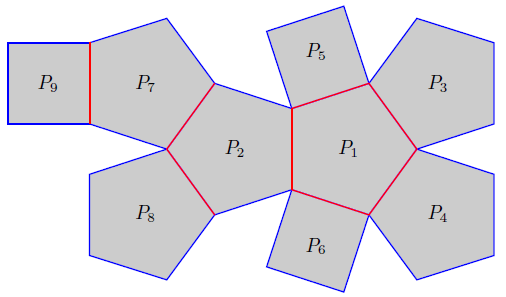# Folding nets into Johnson solids

How does one produce a net for the broadest class of polyhedra?Start with a collection of regular polygons — equilateral triangles, squares, pentagons, etc. Can this collection be glued together to make a solid? For instance, six squares can be fused to make a cube. Norman W. Johnson, a retired mathematician from Wheaton College (Massachusetts) who passed away in 2017, answered this question in the 1960’s: there are the five Platonic solids (eg cube, icosohedron), the thirteen Archimedean solids (eg the truncated icosahedron), the prisms and anti-prisms, and only 92 other solids that are now called the “Johnson solids”. (This fact was later proven by Victor Zalgaller.) All of these solids can be represented as nets, which are two-dimensional designs made up of the faces.

Nets can be folded into three-dimensional solids called polyhedra. (See above for an example of a truncated octahedron.) Given a net of a polyhedron, Reuben Wattenhofer, an undergraduate student who worked with the author, recently created a method to automatically fold many nets into a three-dimensional polyhedron. In this article, we will explore these topics as well as a connection to Eugenia Cheng’s first book.

## Introducing the Johnson solids

There are some interesting facts about the 92 Johnson solids. First, the only regular faces that we can use are triangles, squares, pentagons, hexagons, octagons, and decagons. Five of the solids use only triangles for faces, while 24 use only triangles and squares. Looking at each vertex of a polyhedron, the sum of the angles of the faces that meet there must be less than $360^\circ$, so at most five faces can meet at any vertex. The Johnson solids have some fascinating name such as the elongated pentagonal cupola, the square gyrobicupola, and the tridiminished icosahedron.The net for the associahedron described in Eugenia Cheng’s book. Folding occurs along the red edges.

Wattenhofer’s algorithms, written in Mathematica, create animations of foldings as well as files that can be used for 3D printing of the solids. A key idea behind the code is that for any net, we observe that the inner edges where the polygons are joined together are axes for folding, while the outer edges can be paired off by how they will be glued together. (See above for an example, and note that there are an even number of blue edges.) Once the pairings are determined, the mathematical question is to determine how far to fold the faces so that they meet.

In the main algorithm, two faces — such as $P_3$ and $P_5$ in the above — are rotated until their paired edges join together. This is done by defining the distance between points $K$ and $K’$ as a function of the rotation angles of the two faces and then minimizing that function using numerical multivariable calculus. (Sometimes this is done by holding one of the faces frozen and rotating the other face.) There are some complications in certain situations, such as points where five edges come together. These “degree five vertices” can be problematic, and Wattenhofer’s code tries to rearrange the net to reduce the offending vertex’s degree. Ultimately, though, for most of the Johnson solids, the algorithms do what they are supposed to do, creating an animated folding gif as well as an STL (stereolithography) file that can be used for 3D printing.

The initial motivation for this project was a discussion of the folding of nets in Eugenia Cheng’s first book How To Bake $\pi$: An Edible Exploration of the Mathematics of Mathematics. In that book, she presents the associahedron net and writes the following:

“Just don’t try to make it out of thick card stock, because it won’t quite fit together — it needs to be made from paper that’s a bit bendy, otherwise the pentagon and squares would have to be a bit wonky for them all to fit together.”

Why doesn’t Cheng’s net fold together properly? It is made up six regular pentagons and three squares, the type of polyhedron that Johnson was interested in. However, none of the 92 solids on Johnson’s list is made up of six pentagons and three squares! So, the only way that the polygons in Cheng’s net will fit together is if you warp some of them, leading to a “wonky” solid.Edward Aboufadel is a professor of mathematics at Grand Valley State University, in western Michigan. To learn about his other applied mathematics projects: https://sites.google.com/site/aboufadelreu/